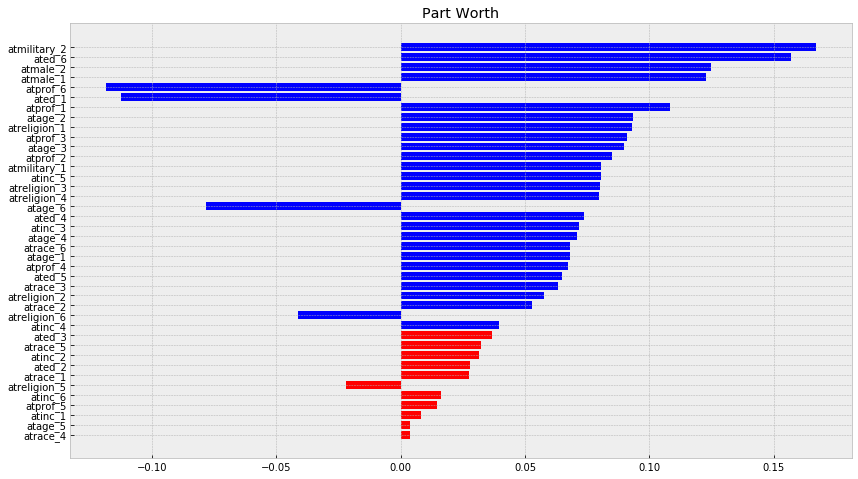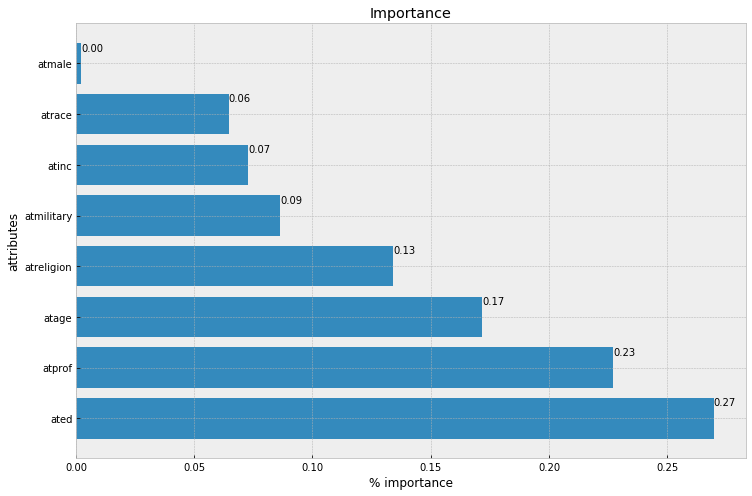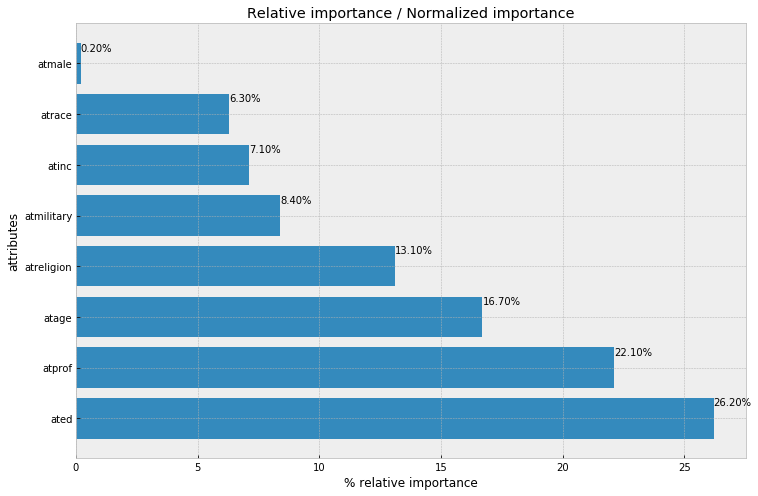# How to Do Conjoint Analysis in python

This post shows how to do conjoint analysis using python. Conjoint analysis is a method to find the most prefered settings of a product .

Usual fields of usage :

• Marketing
• Product management
• Operation Research

For example:

• testing customer acceptance of new product design.
import pandas as pd
import numpy as np


Here we used Immigrant conjoint data described by . It consists of 2 possible conjoint methods: choice-based conjoint (with selected column as target variable) and rating-based conjoint (with rating as target variable).

# Preparing The Data

# taken from imigrant conjoint data

df.head()

resID atmilitary atreligion ated atprof atinc atrace atage atmale selected rating
0 383 1 6 3 6 6 1 6 2 0 0.333333
1 383 2 1 1 4 3 6 4 1 1 0.500000
2 383 1 3 5 5 1 2 5 2 1 0.666667
3 383 2 4 5 3 2 1 6 1 0 0.666667
4 383 2 1 2 3 6 2 2 2 0 0.333333
# checking for empty data
df.isnull().sum()

resID          0
atmilitary     0
atreligion     0
ated           0
atprof         0
atinc          0
atrace         0
atage          0
atmale         0
selected       0
rating        10
dtype: int64

# remove empty data
clean_df = df[~df.rating.isnull()]


# Doing The Conjoint Analysis


y = clean_df['selected']
x = clean_df[[x for x in df.columns if x != 'selected' and x != 'resID' and x != 'rating']]

xdum = pd.get_dummies(x, columns=[c for c in x.columns if c != 'selected'])

atmilitary_1 atmilitary_2 atreligion_1 atreligion_2 atreligion_3 atreligion_4 atreligion_5 atreligion_6 ated_1 ated_2 ... atrace_5 atrace_6 atage_1 atage_2 atage_3 atage_4 atage_5 atage_6 atmale_1 atmale_2
0 1 0 0 0 0 0 0 1 0 0 ... 0 0 0 0 0 0 0 1 0 1
1 0 1 1 0 0 0 0 0 1 0 ... 0 1 0 0 0 1 0 0 1 0
2 1 0 0 0 1 0 0 0 0 0 ... 0 0 0 0 0 0 1 0 0 1
3 0 1 0 0 0 1 0 0 0 0 ... 0 0 0 0 0 0 0 1 1 0
4 0 1 1 0 0 0 0 0 0 1 ... 0 0 0 1 0 0 0 0 0 1

5 rows × 40 columns

 has complete definition of important attributes in Conjoint Analysis

Utility of an alternative $U(x)$ is

$U(x) = \sum_{i=1}^{m}\sum_{j=1}^{k_{i}}u_{ij}x_{ij}$

where:

$u_{ij}$: part-worth contribution (utility of jth level of ith attribute)

$k_{i}$: number of levels for attribute i

$m$: number of attributes

Importance of an attribute $R_{i}$ is defined as $R_{i} = max(u_{ij}) - min(u_{ik})$ $R_{i}$ is the $i$-th attribute

Relative Importance of an attribute $Rimp_{i}$ is defined as $Rimp_{i} = \frac{R_{i}}{\sum_{i=1}^{m}{R_{i}}}$

Essentially conjoint analysis (traditional conjoint analysis) is doing linear regression where the target variable could be binary (choice-based conjoint analysis), or 1-7 likert scale (rating conjoint analysis), or ranking(rank-based conjoint analysis).

import statsmodels.api as sm
import seaborn as sns
import matplotlib.pyplot as plt
plt.style.use('bmh')

res = sm.OLS(y, xdum, family=sm.families.Binomial()).fit()
res.summary()

Dep. Variable: R-squared: selected 0.091 OLS 0.083 Least Squares 10.72 Sun, 09 Dec 2018 7.39e-51 15:49:37 -2343.3 3456 4753. 3423 4956. 32 nonrobust
coef std err t P>|t| [0.025 0.975] 0.0808 0.008 9.585 0.000 0.064 0.097 0.1671 0.008 19.810 0.000 0.151 0.184 0.0931 0.018 5.132 0.000 0.058 0.129 0.0578 0.018 3.144 0.002 0.022 0.094 0.0803 0.018 4.411 0.000 0.045 0.116 0.0797 0.018 4.326 0.000 0.044 0.116 -0.0218 0.018 -1.185 0.236 -0.058 0.014 -0.0411 0.018 -2.256 0.024 -0.077 -0.005 -0.1124 0.018 -6.115 0.000 -0.148 -0.076 0.0278 0.019 1.464 0.143 -0.009 0.065 0.0366 0.019 1.942 0.052 -0.000 0.074 0.0737 0.018 4.076 0.000 0.038 0.109 0.0649 0.018 3.570 0.000 0.029 0.101 0.1572 0.018 8.949 0.000 0.123 0.192 0.1084 0.018 5.930 0.000 0.073 0.144 0.0852 0.019 4.597 0.000 0.049 0.122 0.0910 0.018 5.060 0.000 0.056 0.126 0.0674 0.018 3.716 0.000 0.032 0.103 0.0145 0.019 0.779 0.436 -0.022 0.051 -0.1186 0.018 -6.465 0.000 -0.155 -0.083 0.0081 0.018 0.448 0.654 -0.027 0.043 0.0316 0.019 1.662 0.097 -0.006 0.069 0.0716 0.018 4.020 0.000 0.037 0.106 0.0397 0.018 2.154 0.031 0.004 0.076 0.0808 0.018 4.451 0.000 0.045 0.116 0.0161 0.018 0.872 0.383 -0.020 0.052 0.0274 0.018 1.494 0.135 -0.009 0.063 0.0527 0.018 2.881 0.004 0.017 0.089 0.0633 0.018 3.556 0.000 0.028 0.098 0.0037 0.019 0.198 0.843 -0.033 0.040 0.0324 0.018 1.787 0.074 -0.003 0.068 0.0683 0.019 3.687 0.000 0.032 0.105 0.0680 0.018 3.770 0.000 0.033 0.103 0.0934 0.019 4.957 0.000 0.056 0.130 0.0900 0.018 4.967 0.000 0.054 0.125 0.0711 0.019 3.837 0.000 0.035 0.107 0.0038 0.018 0.208 0.835 -0.032 0.039 -0.0783 0.018 -4.276 0.000 -0.114 -0.042 0.1228 0.008 14.616 0.000 0.106 0.139 0.1250 0.008 14.787 0.000 0.108 0.142
 Omnibus: Durbin-Watson: 0.07 2.872 0.966 391.306 -0.011 1.07e-85 1.352 1.27e+16

Warnings:
 Standard Errors assume that the covariance matrix of the errors is correctly specified.
 The smallest eigenvalue is 4.28e-29. This might indicate that there are
strong multicollinearity problems or that the design matrix is singular.

df_res = pd.DataFrame({
'param_name': res.params.keys()
, 'param_w': res.params.values
, 'pval': res.pvalues
})
# adding field for absolute of parameters
df_res['abs_param_w'] = np.abs(df_res['param_w'])
# marking field is significant under 95% confidence interval
df_res['is_sig_95'] = (df_res['pval'] < 0.05)
# constructing color naming for each param
df_res['c'] = ['blue' if x else 'red' for x in df_res['is_sig_95']]

# make it sorted by abs of parameter value
df_res = df_res.sort_values(by='abs_param_w', ascending=True)

f, ax = plt.subplots(figsize=(14, 8))
plt.title('Part Worth')
pwu = df_res['param_w']
xbar = np.arange(len(pwu))
plt.barh(xbar, pwu, color=df_res['c'])
plt.yticks(xbar, labels=df_res['param_name'])
plt.show()Now we will compute importance of every attributes, with definition from before, where:

$R_{i} = max(u_{ij}) - min(u_{ik})$ $Rimp_{i} = \frac{R_{i}}{\sum_{i=1}^{m}{R_{i}}}$

sum of importance on attributes will approximately equal to the target variable scale: if it is choice-based then it will equal to 1, if it is likert scale 1-7 it will equal to 7. In this case, importance of an attribute will equal with relative importance of an attribute because it is choice-based conjoint analysis (the target variable is binary).

# need to assemble per attribute for every level of that attribute in dicionary
range_per_feature = dict()
for key, coeff in res.params.items():
sk =  key.split('_')
feature = sk
if len(sk) == 1:
feature = key
if feature not in range_per_feature:
range_per_feature[feature] = list()

range_per_feature[feature].append(coeff)

# importance per feature is range of coef in a feature
# while range is simply max(x) - min(x)
importance_per_feature = {
k: max(v) - min(v) for k, v in range_per_feature.items()
}

# compute relative importance per feature
# or normalized feature importance by dividing
# sum of importance for all features
total_feature_importance = sum(importance_per_feature.values())
relative_importance_per_feature = {
k: 100 * round(v/total_feature_importance, 3) for k, v in importance_per_feature.items()
}


alt_data = pd.DataFrame(
list(importance_per_feature.items()),
columns=['attr', 'importance']
).sort_values(by='importance', ascending=False)

f, ax = plt.subplots(figsize=(12, 8))
xbar = np.arange(len(alt_data['attr']))
plt.title('Importance')
plt.barh(xbar, alt_data['importance'])
for i, v in enumerate(alt_data['importance']):
ax.text(v , i + .25, '{:.2f}'.format(v))
plt.ylabel('attributes')
plt.xlabel('% importance')
plt.yticks(xbar, alt_data['attr'])
plt.show()alt_data = pd.DataFrame(
list(relative_importance_per_feature.items()),
columns=['attr', 'relative_importance (pct)']
).sort_values(by='relative_importance (pct)', ascending=False)

f, ax = plt.subplots(figsize=(12, 8))
xbar = np.arange(len(alt_data['attr']))
plt.title('Relative importance / Normalized importance')
plt.barh(xbar, alt_data['relative_importance (pct)'])
for i, v in enumerate(alt_data['relative_importance (pct)']):
ax.text(v , i + .25, '{:.2f}%'.format(v))
plt.ylabel('attributes')
plt.xlabel('% relative importance')
plt.yticks(xbar, alt_data['attr'])
plt.show()